# 贝叶斯定理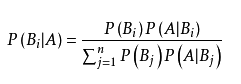P(Bi)P(A|Bi)是联合概率P(A Bi)，表示事件 A 和事件 Bi 同时发生的概率
nj=1P(Bj)P(A|Bj)的结果为随机事件 A 发生的概率

# 朴素贝叶斯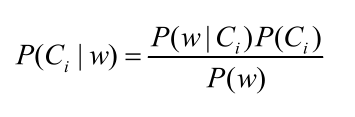P(w|Ci)的计算：
w 是特征向量，若将其展开，则可将P(w|Ci)写作P(w0,w1,w2,…,wn|Ci)，由特征条件独立的假设，即有如下运算

P(w|Ci) = P(w0|Ci)P(w1|Ci)P(w2|Ci)…P(wn|Ci)

# 源代码

# naive_bayes.py  -- 朴素贝叶斯

import math

class NB():
def __init__(self):
self.cla_all_num = 0
self.cla_num = {}
self.cla_tag_num = {}
self.landa = 1  # 拉普拉斯修正值

def train(self, taglist, cla):  # 训练，每次插入一条数据
# 插入分类
self.cla_all_num += 1
if cla in self.cla_num:  # 是否已存在该分类
self.cla_num[cla] += 1
else:
self.cla_num[cla] = 1
if cla not in self.cla_tag_num:
self.cla_tag_num[cla] = {}  # 创建每个分类的标签字典
# 插入标签
tmp_tags = self.cla_tag_num[cla]  # 浅拷贝，用作别名
for tag in taglist:
if tag in tmp_tags:
tmp_tags[tag] += 1
else:
tmp_tags[tag] = 1

def P_C(self, cla):  # 计算分类 cla 的先验概率
return self.cla_num[cla] / self.cla_all_num

def P_all_C(self):  # 计算所有分类的先验概率
tmpdict = {}
for key in self.cla_num.keys():
tmpdict[key] = self.cla_num[key] / self.cla_all_num
return tmpdict

def P_W_C(self, tag, cla):  # 计算分类 cla 中标签 tag 的后验概率
tmp_tags = self.cla_tag_num[cla]  # 浅拷贝，用作别名
if tag not in self.cla_tag_num[cla]:
return self.landa / (self.cla_num[cla] + len(tmp_tags) * self.landa)  # 拉普拉斯修正
return (tmp_tags[tag] + self.landa) / (self.cla_num[cla] + len(tmp_tags) * self.landa)

def test(self, test_tags):  # 测试
res = ''
res_P = None
for cla in self.cla_num.keys():
log_P_W_C = 0
for tag in test_tags:
log_P_W_C += math.log(self.P_W_C(tag, cla))
tmp_P = log_P_W_C + math.log(self.P_C(cla))  # P(w|Ci) * P(Ci)
if res_P is None:
res = cla
res_P = tmp_P
if tmp_P > res_P:
res = cla
res_P = tmp_P
return res

def set_landa(self, landa):
self.landa = landa

def clear(self):  # 重置模型
self.cla_all_num = 0
self.cla_num.clear()
self.cla_tag_num.clear()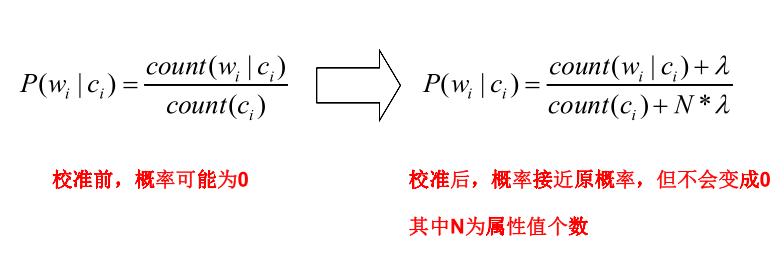def P_W_C(self, tag, cla):  # 计算分类 cla 中标签 tag 的后验概率
tmp_tags = self.cla_tag_num[cla]  # 浅拷贝，用作别名
if tag not in self.cla_tag_num[cla]:
return self.landa / (self.cla_num[cla] + len(tmp_tags) * self.landa)  # 拉普拉斯修正
return (tmp_tags[tag] + self.landa) / (self.cla_num[cla] + len(tmp_tags) * self.landa)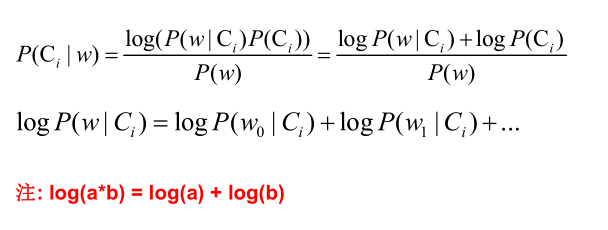for cla in self.cla_num.keys():
log_P_W_C = 0
for tag in test_tags:
log_P_W_C += math.log(self.P_W_C(tag, cla))
tmp_P = log_P_W_C + math.log(self.P_C(cla))  # P(w|Ci) * P(Ci)


# 样例测试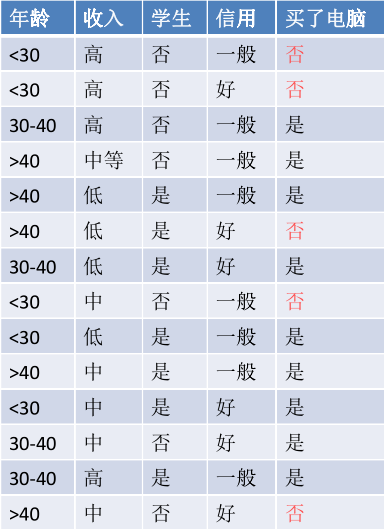if __name__ == '__main__':
nb = NB()  # 生成模型
# 训练模型
# 年龄，收入，是否学生，信用等级  --->  是否买了电脑
nb.train(['<30', '高', '否', '一般'], '否')
nb.train(['<30', '高', '否', '好'], '否')
nb.train(['30-40', '高', '否', '一般'], '是')
nb.train(['>40', '中', '否', '一般'], '是')
nb.train(['>40', '低', '是', '一般'], '是')
nb.train(['>40', '低', '是', '好'], '否')
nb.train(['30-40', '低', '是', '好'], '是')
nb.train(['<30', '中', '否', '一般'], '否')
nb.train(['<30', '低', '是', '一般'], '是')
nb.train(['>40', '中', '是', '一般'], '是')
nb.train(['<30', '中', '是', '好'], '是')
nb.train(['30-40', '中', '否', '好'], '是')
nb.train(['30-40', '高', '是', '一般'], '是')
nb.train(['>40', '中', '否', '好'], '否')



	# 测试模型
testdata = ['<30', '中', '是', '一般']
print('测试结果：', nb.test(testdata))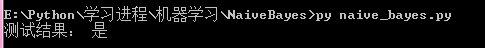w = ( 年龄 <30，收入中等，是学生，信用一般 )
P(w|Ci) = P(w0|Ci) ) * P(w1|Ci) * P(w2|Ci) * P(w3|Ci)

P( 买了电脑 ) = 9/14 = 0.643
P( 年龄<30 | 买了电脑 ) = 2/9 = 0.222
P( 收入中等 | 买了电脑 ) = 4/9 = 0.444
P( 是学生 | 买了电脑 ) = 6/9 = 0.667
P( 信用一般 | 买了电脑 ) = 6/9 = 0.667
P( w | 买了电脑 ) = 0.222 * 0.444 * 0.667 * 0.667 = 0.044
P( w | 买了电脑 ) * P( 买了电脑 ) = 0.044 * 0.643 = 0.028

P( 未买电脑 ) = 5/14 = 0.357
P( 年龄<30 | 未买电脑 ) = 3/5 = 0.600
P( 收入中等 | 未买电脑 ) = 2/5 = 0.400
P( 是学生 | 未买电脑 ) = 1/5 = 0.200
P( 信用一般 | 未买电脑 ) = 2/5 = 0.400
P( w | 未买电脑 ) = 0.6 * 0.4 * 0.2 * 0.4 = 0.019
P( w | 未买电脑 ) * P( 未买电脑 ) = 0.019 * 0.357 = 0.007

P( 买了电脑 | w ) = P( w | 买了电脑 ) * P( 买了电脑 ) / P(w) = 0.028 / P(w)
P( 未买电脑 | w ) = P( w | 未买电脑 ) * P( 未买电脑 ) / P(w) = 0.007 / P(w)

09-094349

#### Python3：《机器学习实战》之朴素贝叶斯（1）算法概述01-121083

#### 朴素贝叶斯（附python3代码）

07-0876

#### python3__机器学习__朴素贝叶斯分类

03-315594

#### python实践之：用朴素贝叶斯实现分类

01-241038

#### 机器学习-朴素贝叶斯(python3代码实现)

07-141001

#### 机器学习实战（3）——朴素贝叶斯（上）（附带Python3源码与资源分享）

03-27133

#### NLP_Python3——朴素贝叶斯算法

03-13157

#### 朴素贝叶斯（手撕代码Python3）

01-08110

#### JS简单实现朴素贝叶斯分类器©️2020 CSDN 皮肤主题: 书香水墨 设计师: CSDN官方博客点击重新获取扫码支付1.余额是钱包充值的虚拟货币，按照1:1的比例进行支付金额的抵扣。
2.余额无法直接购买下载，可以购买VIP、C币套餐、付费专栏及课程。余额充值